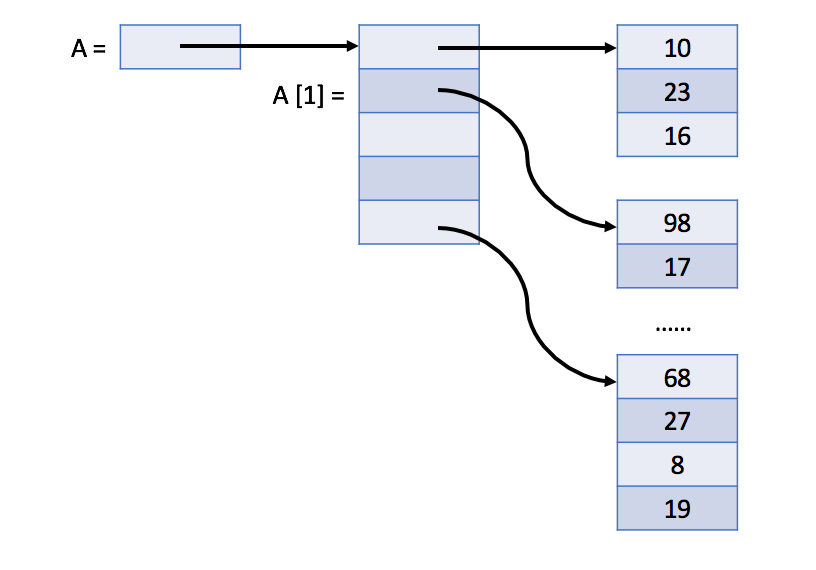# Array

## Array Concepts

### Array

An `array` is a basic data structure to `store a collection of elements sequentially` . But elements can be `accessed randomly` since each element in the array can be identified by an array `index`.

### Dynamic Array

... an array has a fixed capacity and we need to specify the size of the array when we initialize it. Sometimes this will be somewhat inconvenient and wasteful. Most programming languages offer built-in dynamic array which is still a random access list data structure but with variable size. For example, we have vector in C++ and ArrayList in Java.
Operations in Dynamic Array
// "static void main" must be defined in a public class.
public class Main {
public static void main(String[] args) {
// 1. initialize
List<Integer> v0 = new ArrayList<>();
List<Integer> v1; // v1 == null
// 2. cast an array to a vector
Integer[] a = {0, 1, 2, 3, 4};
v1 = new ArrayList<>(Arrays.asList(a));
// 3. make a copy
List<Integer> v2 = v1; // another reference to v1
List<Integer> v3 = new ArrayList<>(v1); // make an actual copy of v1
// 3. get length
System.out.println("The size of v1 is: " + v1.size());
// 4. access element
System.out.println("The first element in v1 is: " + v1.get(0));
// 5. iterate the vector
System.out.print("[Version 1] The contents of v1 are:");
for (int i = 0; i < v1.size(); ++i) {
System.out.print(" " + v1.get(i));
}
System.out.println();
System.out.print("[Version 2] The contents of v1 are:");
for (int item : v1) {
System.out.print(" " + item);
}
System.out.println();
// 6. modify element
v2.set(0, 5); // modify v2 will actually modify v1
System.out.println("The first element in v1 is: " + v1.get(0));
v3.set(0, -1);
System.out.println("The first element in v1 is: " + v1.get(0));
// 7. sort
Collections.sort(v1);
// 8. add new element at the end of the vector
// 9. delete the last element
v1.remove(v1.size() - 1);
}
}

## 2D Array - Matrix

In some languages, the multidimensional array is actually implemented internally as a one-dimensional array while in some other languages, there is actually no multidimensional array at all.
1. C++ stores the two-dimensional array as a one-dimensional array.
The picture below shows the actual structure of an `M * N` array A:So actually `A[i][j]` equals to `A[i * N + j]` if we defined_A_as a one-dimensional array which also contains `M * N` elements.
2. In Java, the two-dimensional array is actually a one-dimensional array which contains M elements, each of which is an array of N integers.
The picture below shows the actual structure of a two-dimensional array A in Java:## Array Basic Operations

### Iterate Through Loop in Java

package crunchify.com.tutorial;
import java.util.ArrayList;
import java.util.Iterator;
import java.util.List;
/**
* @author Crunchify.com
*/
public class CrunchifyIterateThroughList {
public static void main(String[] argv) {
// create list
List<String> crunchifyList = new ArrayList<String>();
// add 4 different values to list
// iterate via "for loop"
System.out.println("==> For Loop Example.");
for (int i = 0; i < crunchifyList.size(); i++) {
System.out.println(crunchifyList.get(i));
}
// iterate via "New way to loop"
for (String temp : crunchifyList) {
System.out.println(temp);
}
// iterate via "iterator loop"
System.out.println("\n==> Iterator Example...");
Iterator<String> crunchifyIterator = crunchifyList.iterator();
while (crunchifyIterator.hasNext()) {
System.out.println(crunchifyIterator.next());
}
// iterate via "while loop"
System.out.println("\n==> While Loop Example....");
int i = 0;
while (i < crunchifyList.size()) {
System.out.println(crunchifyList.get(i));
i++;
}
// collection stream() util: Returns a sequential Stream with this collection as its source
System.out.println("\n==> collection stream() util....");
crunchifyList.forEach((temp) -> {
System.out.println(temp);
});
}
}

### Conversion between Array and ArrayList in Java

#### From `Array` to `ArrayList`

// java.util.ArrayList
Element[] array = {new Element(1),new Element(2),new Element(3)};
// 1. Most popular and accepted answer
ArrayList<Element> arrayList = new ArrayList<Element>(Arrays.asList(array));
List<Element> list = Arrays.asList(array);
// Cons: It is not the best, because the size of the list returned from asList() is fixed

#### From `ArrayList` to `Array`:

// Method 1: Using Object[] toArray() method
// (Preferred) Method 2: Using T[] toArray(T[] a)
// a − This is the array into which the elements of the list are to be stored,
// if it is big enough; otherwise, a new array of the same runtime type is allocated for this purpose.
// java.util.ArrayList.toArray(T[])
ArrayList<Integer> foo = new ArrayList<Integer>();
Integer[] bar = foo.toArray(new Integer[foo.size()]);
System.out.println("bar.length = " + bar.length);
// Method 3: Using get() in for loop manually
For example, suppose that`c`is a`Collection`. The following snippet dumps the contents of`c`into a newly allocated array of`Object`whose length is identical to the number of elements in`c`.
Object[] a = c.toArray();
Suppose that`c`is known to contain only strings (perhaps because`c`is of type`Collection<String>`). The following snippet dumps the contents of`c`into a newly allocated array of`String`whose length is identical to the number of elements in`c`.
String[] a = c.toArray(new String);

### Sliding Window

A sliding window is an abstract concept commonly used in array/string problems. A window is a range of elements in the array/string which usually defined by the start and end indices, i.e. `[i,j)` (left-closed, right-open). A sliding window is a window "slides" its two boundaries to the certain direction. For example, if we slide `[i, j)`to the right by 11 element, then it becomes `[i+1, j+1)` (left-closed, right-open).

### Prefix Sum

Prefix sum 数组的 local / global 通用模板，求 min / max 皆可，使用时需要注意初始条件以及顺序:
int[] leftMax = new int[n];
int prefixSum = 0;
// 代表从起始位置开始，值最小的连续和数组
int localMin = 0;
int globalMax = Integer.MIN_VALUE;
for(int i = 0; i < n; i++){
prefixSum += nums[i];
globalMax = Math.max(globalMax, prefixSum - localMin);
localMin = Math.min(localMin, prefixSum);
leftMax[i] = globalMax;
}

Kadane's Algorithm 相比 prefix sum 的特点是，必须要以 nums 做初始化，从 index = 1 开始搜，优点是在处理 prefix product 的时候更容易处理好“-1” 和 “0” 的情况。

public class Solution {
public int maxSubArray(int[] nums) {
if(nums == null || nums.length == 0) return 0;
int localMax = nums;
int globalMax = nums;
for(int i = 1; i < nums.length; i++){
localMax = Math.max(nums[i], localMax + nums[i]);
globalMax = Math.max(globalMax, localMax);
}
return globalMax;
}
}
*Subarray Sum 系列问题参考：